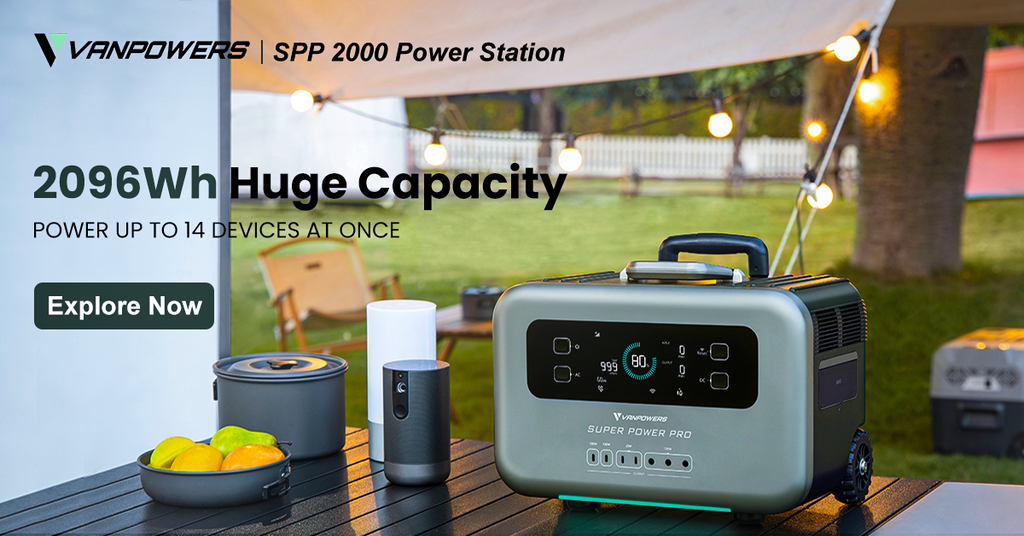## Buyer's Guide: Voltage, Current, and Power

Voltage, current, and power are the basic concepts of electricity. In this article, we will discuss what they are and how they work.

## What is voltage?

Simply put, voltage = pressure. Voltage is the pressure from a circuit’s power supply, also called electromotive force. Voltage (U) can also be described as the difference in potential energy between two points on a circuit, and it is measured in volts (V).

## What is current?

Current is the flow rate of an electric charge. The symbol for current is I, and the unit is the ampere (A). Current and voltage are the two fundamental measures of electricity. Voltage is the cause, and current is the effect. Any component (lamps, motors, heating elements) that converts electrical energy into other forms of energy (light, rotational motion, heat) uses electrical current.

## What is power?

The work done by a current per unit of time is called electrical power. Simply put, power is a measure of how much energy is used over a period of time. The symbol of power is P, and the unit is the watt, abbreviated as "W." Power is a physical quantity that describes how fast work is done.

Relationship between voltage, current, and power

The power (P) of a direct current is equal to the product of the current (I) and the voltage (U), that is, P = I × U.

In electrical systems, increasing current or voltage results in higher power. Power in a circuit exists only when both voltage and current are present. Because electrical power is the product of voltage and current, whether a circuit has high voltage and low current or low voltage and high current, the power dissipated in the circuit can be the same.